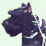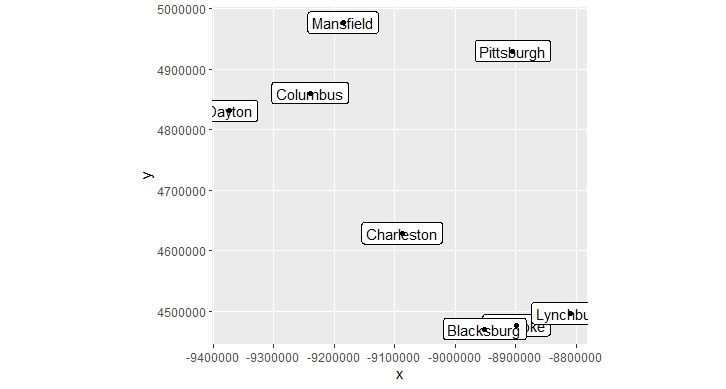# Protocolbuffer Binary Format (PBF) Documentation or Examples?

6258
31
07-17-2019 09:10 AMby
New Contributor III

ArcGIS Enterprise added support for the PBF output format in 10.7.1. We are having difficulty finding any documentation or examples on Esri's implementation of PBF in either the Developer's documentation or Esri's Github outside of Vector Tiles. We would like to look at using this format with some larger datasets. Any pointers would be appreciated?

Tags (1)
31 RepliesNew Contributor II

I don't know what PBF-formatted data ESRI support other than vector tiles and fontstacks. We implemented PBF tile generation by following the MapBox spec (https://docs.mapbox.com/vector-tiles/specification/ and https://github.com/mapbox/vector-tile-spec/blob/master/2.1/vector_tile.proto) and I believe (I didn't implement it myself) taking inspiration from https://github.com/mapbox/vt-pbf. The actual implementation was done in C++ using Google's Protocol Buffers library (https://developers.google.com/protocol-buffers/docs/reference/cpp)by
New Contributor

Is there full documentation on how to de-serialize a vector tile pbf file yet? I hope to take a ESRI vector tile pbf and convert it into geojson. I tried to use the Mapbox spec (https://github.com/mapbox/vector-tile-spec/blob/master/2.1/vector_tile.proto)  but it only transformed the data into another format that I do not know how to handle.

@mgeorge Do you have any update?byEsri Contributor

Hi @JINANYI, we release the FeatureTile Pbf spec here: https://github.com/Esri/arcgis-pbf. The VTL encoding we use is the same as mapbox/vector-tile-spec: Mapbox Vector Tile specification (github.com)

Basically what you will want to do is pull in some pbf parsing library which will then parse the format verbatim. After that, take a look at vector-tile-spec/README.md at master · mapbox/vector-tile-spec (github.com) to see what the properties of the tile mean. I.e., the geometry encoding for mapbox uses command integers that you need to decode what operation is happening.by
New Contributor

I read the references you provided and also this article: FAQ: What is a root tile and how are they used to make a vector tile package with a local coordinate system? https://support.esri.com/en/technical-article/000022396

Using all the info, I tried to decode the a vector tile myself, using World Topographic Map (https://basemaps.arcgis.com/arcgis/rest/services/World_Basemap_v2/VectorTileServer). I downloaded this particular tile in pbf, https://basemaps.arcgis.com/arcgis/rest/services/World_Basemap_v2/VectorTileServer/tile/6/24/17, and start decoding in R language (sorry it's the only language I know).

However, as it turned out, for some unknown reason, the origin of the selected tile seems to be the bottom left corner (x axis positive to the right, y axis positive upward), instead of top left corner. It is shown in the following codes:

`````````{r}
library(sf)
librart(ggplot2)

#the st_read() function automatically uses `MVT' driver, and convert the geometry data into tile coordinates
test <- st_read("17.pbf", layer = "City small scale")

#function that convert tile coordinates into the real mapping coordinates (EPSG: 3857)
shift_tile_coord <- function(sf, level, row, column, crs = 3857) {
if(crs != 3857) stop("currently only supports EPSG:3857 Web Mercator projection")

origin_x <- -20037508.342787
origin_y <- 20037508.342787
maxscale <- 2.958287637957775E8
tile_size <- 512 #pixels of a row/ column
dpi <- 96
inch2mapUnit <- 39.3700787  #1 meter = 39.3700787 inch
tile_extent <- 4096

root_tile_width <- maxscale * (tile_size/(dpi * inch2mapUnit))
selected_tile_width <- root_tile_width/2^level

sf_shifted <- sf

#x = tile_x_coor*selected_tile_width/tile_extent + origin_x + selected_tile_width*column
#y = tile_y_coor*selected_tile_width/tile_extent + origin_y - selected_tile_width*(row + 1)
sf_shifted\$geometry <- sf\$geometry * matrix(c(selected_tile_width/tile_extent, selected_tile_width/tile_extent), ncol = 2)
sf_shifted\$geometry <- sf_shifted\$geometry + matrix(c(origin_x + selected_tile_width * column, origin_y - selected_tile_width * (row + 1)), ncol = 2)

sf_shifted <- st_set_crs(sf_shifted, crs)

return(sf_shifted)
}

#visulaize the data
test_shifted <- shift_tile_coord(test, level = 6, row = 24, column = 17)

ggplot(data = test_shifted) +
geom_sf_label(aes(label = X_name_en)) +
geom_sf() +
coord_sf(datum = st_crs(3857))
`````````Test Data

If you use map viewer to check (https://www.arcgis.com/apps/mapviewer/index.html?layers=7dc6cea0b1764a1f9af2e679f642f0f5#), the coordinates (in Web Mercator, EPSG: 3857) are correct. However, the conversion of a y coordinate = tile_y_coor*selected_tile_width/tile_extent + origin_y - selected_tile_width*(row + 1). This means a y coordinate first starts at the bottom of the tile (thus (row + 1)), and moves upward (positive sign in tile_y_coor*selected_tile_width/tile_extent). This seems to be against the documentation you provided. Is it designed to be this way, or is there something wrong?byEsri Contributor

@JINANYI not sure that I understand your repro. The coordinates after you convert them back to map space have positive y up? That would be expected. Positive y being downward is only in tile coordinate space (0-4096 for VectorTiles).by
New Contributor

@mgeorge I am saying the tile coordinate space of the selected tile (level = 6, row = 24, column = 7), has positive y upward, but I expect positive y downwardby
New Contributor

@mgeorge y coordinate (in Web Mercader projection) = tile_y_coor (0-4096 for tile coordinate) *selected_tile_width/tile_extent + origin_y (in Web Mercader projection) - selected_tile_width*(row + 1)

selected_tile_width, tile_extent are in meter (Web Mercader projection length unit)

row is the row index, 0-63 for level 6 tiles.

Here is the demonstration image (sorry, I tried my best). As you can see, for the selected tile, the origin is at the bottom left cornerbyEsri Contributor

Ok I think I see what you are asking. Basically you are wondering why you don't need to flip the y coordinate of the geometries parsed by the st_read method of the R library you are using?by
New Contributor

@mgeorge Yes, to be precise, I would expect (be aware of the negative sign and changing from "row+1" to "row"):

y coordinate = - tile_y_coor*selected_tile_width/tile_extent + origin_y - selected_tile_width*row